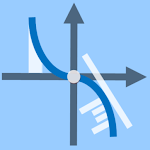100+

# Graphing Calculator - Calculus APK

Version: 1.02 code 3

Developer: A.M.I.R

Released: 2022-04-15

Updated: 2022-06-23## The description of Graphing Calculator - Calculus APK

Free function graphing calculator, parametric equations grapher, point set plotter and complex number calculator for Android, multiple powerful calculators in one easy-to-use integrated scientific graphing calculator.

• Graphs multiple functions, parametric equations and point sets on the same coordinate system.
• Cartesian and polar graphs of the above.
NOTE: to graph the expression in focus press the ‘graph’ button. To graph one or more selected expressions simultaneously, press the ‘Graph’ (capitalized) button OR long press the ‘graph’ button on smaller screens.

• Solve f(x) = 0 to calculate all the x-intercepts of the graph of a function on an interval with a single click.
• Calculate the points of intersection of the graphs of two functions.

This calculator is designed specifically to carry out the most common tasks in calculus as follows

• Calculate symbolic first & second derivatives of functions and graph them.
• Calculate symbolic 1st & 2nd derivatives of parametric equations and graph them.
• Calculate definite integral of functions and parametric curves.
• Calculate area under (or enclosed by) the graphs of functions (or parametric curves) in both the Cartesian and polar cases.
• Calculate arc length of the graphs of functions and parametric curves in both the Cartesian and polar cases.

NOTE: When graphing points you can easily find and graph the polynomial function of the least degree passing through all the points. In addition, you can find and graph the best straight line (linear regression line) that fits a set of points according to Gauss' Least Squares Criteria.

This integrated scientific/graphing calculator also features the following capabilities

• Calculate complex numbers in standard, polar or any other forms.
• Display a table of values for functions and parametric equations even with complex number expressions.
• The Calculator optionally displays the results in scientific, engineering and fixed point notations.
• Calculate relevant expressions in degrees or radians mode.
• Units converter (Time, Mass, Length, Velocity, and many more), and list of constants from various fields of sciences which can be used in your calculations.

In general, calculate or graph mathematical expressions containing standard & advanced mathematical operations and functions including

• Basic operations
• Power functions, x^r and E(x)
• Trigonometric functions and their inverses
• Hyperbolic functions and their inverses
• Exponential and logarithmic functions
• Factorial, the Gamma, Γ, and Psi, Ψ, functions
• Absolute value and step functions, round, floor, ceil

Detailed built-in instruction is provided within the app.

NOTE: You will need Internet connection only when using graph animators which show how graph of functions are created in the polar coordinate system and how parametric graphs are created in either the Cartesian or polar coordinate systems by progressively drawing the corresponding graphs.
___________________
Satisfaction Guaranteed – 100% Refund: If you are not satisfied with your purchase you can request a full refund by emailing the developer (just write "Refund", and state your purchase number and date of purchase) within a week from the time of the purchase (and before the end of the month you made the purchase). A full refund will be asked to be issued by Google Play once your email is received.

Update on
2022-06-23
Package name
com.calcamir.graphingCalculator
Author
A.M.I.R
Version
1.02
Installs
100++
File size
1.418.389 bytes
Category
TOOLS
Requirements
Android 5.0+
Content rating
Rated for 0+
We use cookies and other technologies on this website to enhance your user experience.